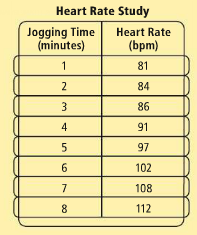#### MAT-HS.S-ID.07

 MAT-HS Targeted Standards(S) Concept: Statistics and Probability(ID) Domain: Interpreting Categorical and Quantitative DataCluster: Interpret linear models MAT-HS.S-ID.07 Interpret the slope (rate of change) and the intercept (constant term) of a linear model in the context of the data.

## Student Learning Targets:

### Knowledge Targets

• I can identify Cartesian coordinate system.
• I can define slope.
• I can recall slope intercept, point-slope, and standard form.
• I know the midpoint and slope formula.

### Reasoning Targets

• I can explain the meaning of the slope and y-intercept in context.

### Skills (Performance) Targets

• I can find slope give Cartesian coordinates, graph, or equation.

• I can
• I can

## Proficiency Scale

 Score Description Sample Activity 4.0 In addition to Score 3.0, the student demonstrates in-depth inferences and applications regarding more complex material that go beyond end of instruction expectations. - 3.5 In addition to Score 3.0 performance, the student demonstrates in-depth inferences and applications regarding the more complex content with partial success. 3.0 The student: can explain the meaning of the slope of a linear model in context can explain the y-intercept of a linear model in context can use a trend line and line of best fit to make predictions The student exhibits no major errors or omissions. Use the following table to estimate the heart rate prior to jogging and after jogging for 16 minutes. Explain why your answer makes sense.2.5 The student demonstrates no major errors or omissions regarding the simpler details and processes (Score 2.0 content) and partial knowledge of the more complex ideas and processes (Score 3.0 content). 2.0 There are no major errors or omissions regarding the simpler details and processes as the student:The student can: can recognize and recall terminology such as: equation, slope, y-intercept can graph a linear model, but can not interpret the results However, the student exhibits major errors or omissions regarding the more complex ideas and processes. - 1.5 The student demonstrates partial knowledge of the simpler details and processes (Score 2.0 content) but exhibits major errors or omissions regarding the more complex ideas and procedures (Score 3.0 content). 1.0 With help, the student demonstrates a partial understanding of some of the simpler details and processes (Score 2.0 content) and some of the more complex ideas and processes (Score 3.0 content). - 0.5 With help, the student demonstrates a partial understanding of some of the simpler details and processes (Score 2.0 content) but not the more complex ideas and processes (Score 3.0 content). 0.0 Even with help, the student demonstrates no understanding or skill. -

## Resources

 Web Vocab